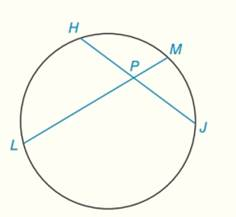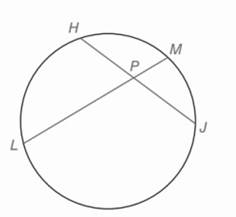Chapter 6.CT, Problem 11CTElementary Geometry For College St...

7th Edition
Alexander + 2 others
ISBN: 9781337614085

Solutions

Chapter
SectionElementary Geometry For College St...

7th Edition
Alexander + 2 others
ISBN: 9781337614085
Textbook Problem

a) If H P = 4 , P J = 5 , and P M = 2 , find L P .b) If H P = x + 1 , P J = x − 1 , L P = 8   , and   P M = 3 ​ , find x .To determine

a)

To find:

LP from,.

Explanation

Given:

HJ and LM are two chord lines intersect inside the circle.

HP=4, PJ=5, and PM=2.

Theorem used:

“If two chords intersect within a circle then the product of the lengths of the segments (parts) of one chord is equal to the product of the lengths of the segments of the other chord”.

Calculation:

Consider the two intersected chords HJ and LM.

From the theorem it is clear that,

HPPJ=PMLP(

To determine

b)

To find:

x in HP=x+1, PJ=x1, LP=8, and PM=3.

Still sussing out bartleby?

Check out a sample textbook solution.

See a sample solution

The Solution to Your Study Problems

Bartleby provides explanations to thousands of textbook problems written by our experts, many with advanced degrees!

Get Started

Find more solutions based on key concepts